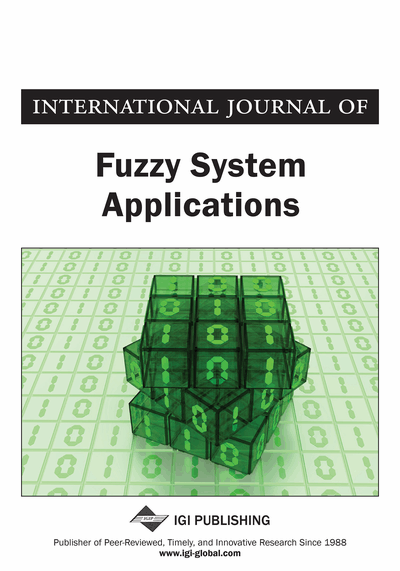# On Generalized Fuzzy Entropy and Fuzzy Divergence Measure with Applications

Surender Singh, Sonam Sharma
DOI: 10.4018/IJFSA.2019070102
OnDemand:
(Individual Articles)
Available
\$37.50
No Current Special Offers

## Abstract

Entropy in a fuzzy set measures the amount of ambiguity/imprecision presented in the fuzzy set. In this article, the authors introduce a generalized fuzzy entropy measure and demonstrate its effectiveness in Multiple Attribute Decision Making (MADM) and superiority from the point of view of structured linguistic variables. This article also introduces a generalized fuzzy directed divergence and investigates its properties. Further, this article demonstrates the effectiveness of the proposed generalized directed divergence in pattern recognition.
Article Preview
Top

## 1. Introduction

The concept of entropy measure is enormously used to measure the amount of uncertainty in a random experiment. Formally, the idea of entropy was proposed by Shannon (1948). In last few decades, enormous work had been reported towards the generalization of probabilistic information measures (For more details, refer to Taneja, 2001). But these measures work only for unambiguous data, i.e., where the data is concerned with a precisely known system.

To deal with ambiguous data/information, Zadeh (1965) introduced fuzzy set theory and incorporated the concept of fuzzy logic to improve upon some shortcomings of binary logic. De Luca and Termini (1972) proposed an axiomatic definition of fuzzy entropy. The entropy of the fuzzy set is considered as the average amount of ambiguity/imprecision associated with that fuzzy set. After De Luca and Termini (1972), many authors proposed the generalization of fuzzy entropy. Kosko (1986) proposed a new fuzzy entropy based on hypercube and distance between fuzzy subset A, nearest vertex Anear and farthest vertex Afar. Hooda (2004), Li and Liu (2008), Bajaj and Hooda (2010) provided parametric generalizations of fuzzy entropy. Verma and Sharma (2011) proposed the generalized exponential fuzzy entropy. Joshi and Kumar (2017b) introduced two parametric exponential fuzzy entropy and many more.

A measure of discrimination between two fuzzy sets is also a significant tool for the study of various engineering problems. Bhandari et al. (1992) proposed the information measure for discrimination between two fuzzy sets. Bhandari and Pal (1993) introduced another discrimination measure which is a special case of entropy proposed by De Luca and Termini (1972). Hooda (2004) provided one parametric generalization of the fuzzy divergence measure of Bhandari et al. (1992). Bhatia and Singh (2012) proposed some generalized fuzzy divergence measures corresponding to some existing probabilistic divergence measures. Bhatia and Singh (2013) had presented an approach using aggregation operators to obtain symmetric divergence and application of fuzzy divergence measure in image segmentation. Most of the work related to the generalization of fuzzy entropy and fuzzy divergence measures did not give justification for preference of the generalized versions of fuzzy entropy and fuzzy divergence measures over classical versions of these fuzzy information measures. In the present work, we study one parametric generalization of De Luca and Termini’ (1972) fuzzy entropy and provide justification for preference of the proposed generalized fuzzy entropy. Further, we also study one parametric generalization of Bhandari et al. (1992) divergence measure and establish its superiority in some problems of pattern recognition. Zhu & Li (2016) derived a new entropy formula concerning the amount of information and reliability in intuitionistic fuzzy sets. Fuzzy entropy and divergence measures are useful in various multi-attribute group decision- making methods such as TOPSIS, MOORA, VIKOR, TODIM, etc. Many authors (Chen & Li, 2010; Riu & Li, 2010; Shemshadi et al., 2011; Awasthi & Kannan, 2016; Ren et al., 2016, and Joshi & Kumar, 2018) have studied these methods using fuzzy/intuitionistic fuzzy/interval-valued intuitionistic fuzzy entropy and divergence measures from various viewpoints.

## Complete Article List

Search this Journal:
Reset
Volume 13: 1 Issue (2024)
Volume 12: 1 Issue (2023)
Volume 11: 4 Issues (2022)
Volume 10: 4 Issues (2021)
Volume 9: 4 Issues (2020)
Volume 8: 4 Issues (2019)
Volume 7: 4 Issues (2018)
Volume 6: 4 Issues (2017)
Volume 5: 4 Issues (2016)
Volume 4: 4 Issues (2015)
Volume 3: 4 Issues (2013)
Volume 2: 4 Issues (2012)
Volume 1: 4 Issues (2011)
View Complete Journal Contents Listing